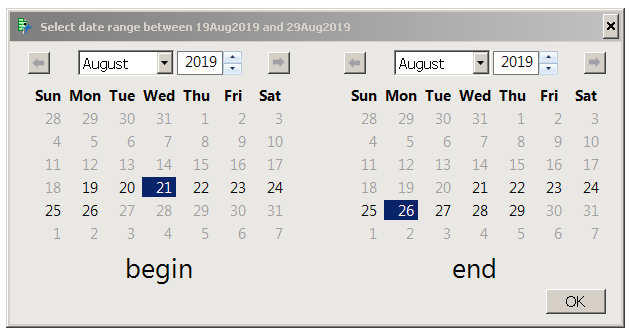Choose Language Hide Translation Bar
Select Date Range with Calendar Boxes

This JSL function displays a modal dialog containing two calendar boxes. The boxes are linked with JSL so picking a minimum date in the left box grays unavailable dates in the right box, and similar for the other direction.Linked calendar boxes

The JSL is written as a function you can call with an optional limit on the range of dates (shown in the title bar). The function call returns a list:

{21Aug2019, 26Aug2019}

``````promptDateRange = Function( {minAllowed = 1jan1001, maxAllowed = 31dec2999},
{roundMidnight, result, startDate, endDate}, // local variables

roundMidnight = Function( {datetime}, // return the date, without the time...which is midnight at the start of a day
As Date( Date MDY( Month( datetime ), Day( datetime ), Year( datetime ) ) )
);
minAllowed = roundMidnight( minAllowed );
maxAllowed = roundMidnight( maxAllowed );
result = New Window( "Select date range between " || Char( minAllowed ) || " and " || Char( maxAllowed ),
modal, // modal: the window must be closed before JMP can continue
// do the validation in OnValidate, NOT OnClose! Doing it in OnClose will prevent canceling, which is Bad!
<<OnValidate( (startDate << getDate) <= (endDate << getDate) ), // validate the range makes sense
// capture answers when the window is closed
<<OnClose( // convert the variables holding the calendar boxes into the value in the calendar box
startDate = roundMidnight( startDate << getDate ); // throw away time part; this rounding
endDate = roundMidnight( endDate << getDate ); // is needed if the default date is unchanged.
1; // return 1: the window will close. Validation was already done.
),
// when the window first opens, remove the Today buttons.
<<OnOpen( // They don't run callback or round to midnight.
(((startDate)[Button Box( 3 )])) << delete;
(((endDate)[Button Box( 3 )])) << delete;
),
H List Box( // make a left/right pair of calendars in a horizontal list box
V List Box(
startDate = Calendar Box(
<<min date( minAllowed ),
<<max date( maxAllowed ),
<<showTime( 0 ), // don't show the time
// make the left (smaller date) box adjust the upper date's lowest possible value
<<setfunction(
Function( {this, date},
{},
endDate << mindate( roundMidnight( startDate << getDate ) );
If( roundMidnight( endDate << getDate ) < (endDate << getMinDate),
endDate << date( endDate << getMinDate )
);
startDate << maxdate( roundMidnight( endDate << getDate ) );
)
)
),
H Center Box( Text Box( "begin", <<setFontSize( 20 ) ) )
),
Spacer Box( size( 50, 1 ) ), // add a bit of space between the calendars
V List Box(
endDate = Calendar Box(
<<min date( minAllowed ),
<<max date( maxAllowed ),
<<showtime( 0 ),  // don't show time
// make the right (bigger date) box adjust the lower date's biggest possible value
<<setfunction(
Function( {this, date},
{},
endDate << mindate( roundMidnight( startDate << getDate ) );
startDate << maxdate( roundMidnight( endDate << getDate ) );
If( roundMidnight( startDate << getDate ) > (startDate << getMaxDate),
startDate << date( startDate << getMaxDate )
);
)
)
),
H Center Box( Text Box( "end", <<setFontSize( 20 ) ) )
)
)
);

If( result["Button"] == 1, // result == {Button( 1 )} if OK is pressed
Eval List( {startDate, endDate} ) // ok
,
{., .} // cancel
);

);

Write( promptDateRange( Today() - In Days( 5 ), Today() + In Days( 5 ) ) );``````

This JSL turns off the time display in the calendar control and removes any seconds after midnight whenever they appear. The JSL also sets a valid range of dates for the left and right controls; picking a date in the left control changes the minimum date in the right control, and similar in the other direction. The today buttons are removed as well. If you try to modify this JSL to support time you'll discover the today buttons don't make the callback and the time they pick is not midnight-rounded like the minimum and maximum date values.